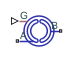# Valve Plate Orifice (IL)

Variable orifice in an isothermal axial-piston machine

Since R2020a

•Libraries:
Simscape / Fluids / Isothermal Liquid / Pumps & Motors / Auxiliary Components

## Description

The Valve Plate Orifice (IL) block models a crescent-shaped opening between moving pistons and a pump in an axial-piston machine. The rotating pistons periodically connect to the pump intake or discharge through the orifice plate. You can connect two valve plate blocks to each cylinder of an axial-piston pump to represent both the pump inlet and outlet slots.

A rotating cylinder, with one crescent slot, is connected to the pump intake at port A and pump discharge at port B. These points are connected to the plate between the Pressure carryover angle and $\pi$ radians (180 degrees) from each other. The plate rotation angle is set by the signal at port G. The cylinder position angle, γ, is the sum of the position signal, G, and the initial angle offset, the Phase angle, γ0:

`$\gamma ={\gamma }_{0}+G.$`

γ is always between 0 and 2π. For any combined signal and offset larger than 2π rad, γ is maintained at 2π, and for any combined signal and offset lower than 0 rad, and γ is maintained at 0. To change the initial position of the orifice relative to the slot, you can adjust the Phase angle parameter.

Axial-Piston Machine With Five PistonsThe numbers in the diagram indicate the components of an axial-piston machine:

1. Valve plate orifice

2. Rotor

3. Piston

4. Driving shaft

5. Swash plate

### Cylinder Angle

The cylinder rotational alignment with the slot is described by the following angles:

• Cylinder angle at rotation onto slot, γ1:

`${\gamma }_{1}=\Psi -\frac{r}{R}$`

• Cylinder angle at complete rotation onto slot, γ2:

`${\gamma }_{2}=\Psi +\frac{r}{R}$`

• Cylinder angle at rotation beyond slot, γ3:

`${\gamma }_{3}=\pi -2\frac{r}{R}$`

• Cylinder angle at complete rotation beyond slot, γ4:

`${\gamma }_{4}=\pi$`

where:

• Ψ is the Pressure carryover angle. This angle represents the average angular distance the piston travels during its pressure transition period from a closed to opened slot.

• r is the half of the Cylinder orifice diameter.

• R is the Cylinder block pitch radius.

### Orifice Opening Area

Orifice area calculations during cylinder motionThe transition opening area, which is the opening between the cylinder rotation angles γ1 and γ2, is calculated as:

`${A}_{{\gamma }_{1}{\gamma }_{2}}={S}_{opening}+{S}_{Leak}={r}^{2}\left(2{\beta }_{opening}-\mathrm{sin}\left(2{\beta }_{opening}\right)\right)+{S}_{Leak}.$`

The transition closing area, which is the opening between the cylinder rotation angles γ3 and γ4, is calculated as:

`${A}_{{\gamma }_{3}{\gamma }_{4}}={S}_{closing}+{S}_{Leak}={r}^{2}\left(2{\beta }_{closing}-\mathrm{sin}\left(2{\beta }_{closing}\right)\right)+{S}_{Leak},$`

where the opening and closing parameters are:

`${\beta }_{opening}={\mathrm{cos}}^{-1}\left(\frac{R}{r}\mathrm{sin}\left(\frac{\left(\psi +\frac{r}{R}\right)-\gamma }{2}\right)\right),$`

and

`${\beta }_{closing}={\mathrm{cos}}^{-1}\left(\frac{R}{r}\mathrm{sin}\left(\frac{\gamma -\left(\pi -2\frac{r}{R}\right)}{2}\right)\right).$`

The area between γ2 and γ3 is ${A}_{{\gamma }_{2}{\gamma }_{3}}={S}_{Max}+{S}_{Leak},$ and the area between γ4 and γ1 is ${A}_{{\gamma }_{4}{\gamma }_{1}}={S}_{Leak}.$The maximum opening of the orifice is ${S}_{Max}=\pi {r}^{2}.$

A nonzero Smoothing factor can provide additional numerical stability when the orifice is in near-closed or near-open position.

### Numerically-Smoothed Orifice Angle

At the orifice slot entrance and exit angles, you can maintain numerical robustness in your simulation by adjusting the block parameter. If the parameter is nonzero, the block smoothly saturates the transition opening angle between γ1 and γ2 and the transition closing angle between γ3 and γ4. For more information, see Numerical Smoothing.

### Mass Flow Rate Equation

The flow through a valve plate orifice is calculated from the pressure-area relationship:

`$\stackrel{˙}{m}={C}_{d}{A}_{orifice}\sqrt{2\overline{\rho }}\frac{\Delta p}{{\left[\Delta {p}^{2}+\Delta {p}_{crit}^{2}\right]}^{1/4}},$`

where:

• Cd is the Discharge coefficient.

• Aorifice is the area open to flow.

• $\overline{\rho }$ is the average fluid density.

• Δp is the pressure drop over the valve, PAPB.

The critical pressure difference, Δpcrit, is the pressure differential associated with the Critical Reynolds number, Recrit, which is the point of transition between laminar and turbulent flow in the fluid:

`$\Delta {p}_{crit}=\frac{\pi \overline{\rho }}{8{A}_{orifice}}{\left(\frac{\nu {\mathrm{Re}}_{crit}}{{C}_{d}}\right)}^{2}.$`

## Ports

### Conserving

expand all

Entry point to the orifice.

Exit point from the orifice.

### Input

expand all

Cylinder rotation angle in radians, specified as a physical signal..

## Parameters

expand all

Pitch radius of the rotating cylinder.

Diameter of the cylinder slot.

Average angular distance the piston travels during the pressure transition period from a closed to opening slot.

Initial plate offset angle. The total angle between the plate and slot is the sum of the Phase angle and the Pressure carryover angle, Ψ.

Sum of all gaps when the valve is in a fully closed position. Any area smaller than this value is maintained at the specified leakage area. This contributes to numerical stability by maintaining continuity in the flow.

Correction factor that accounts for discharge losses in theoretical flows.

Upper Reynolds number limit for laminar flow through the orifice.

Continuous smoothing factor that introduces a layer of gradual change to the flow response when the valve is in near-open or near-closed positions. Set this value to a nonzero value less than one to increase the stability of your simulation in these regimes.

## Version History

Introduced in R2020a